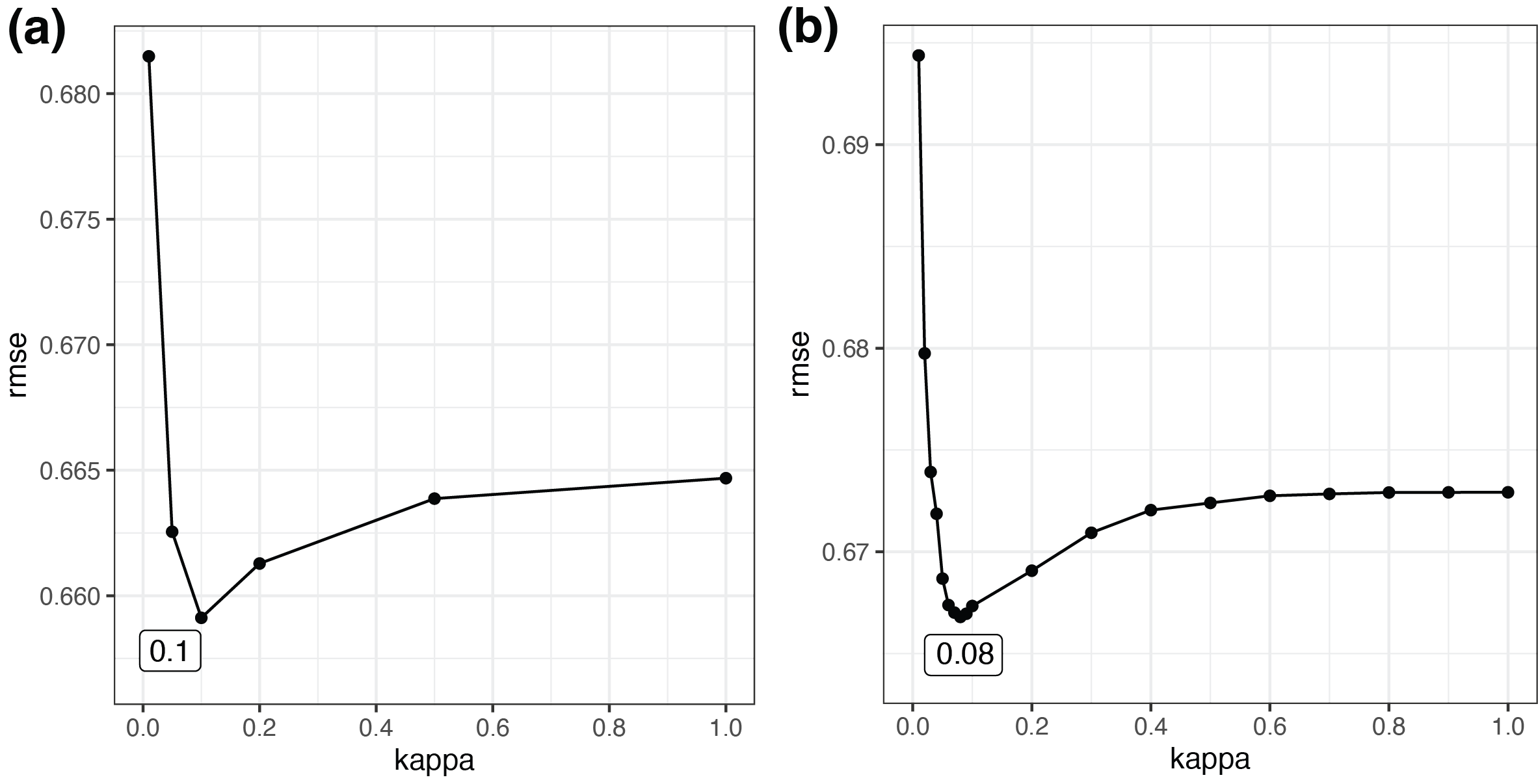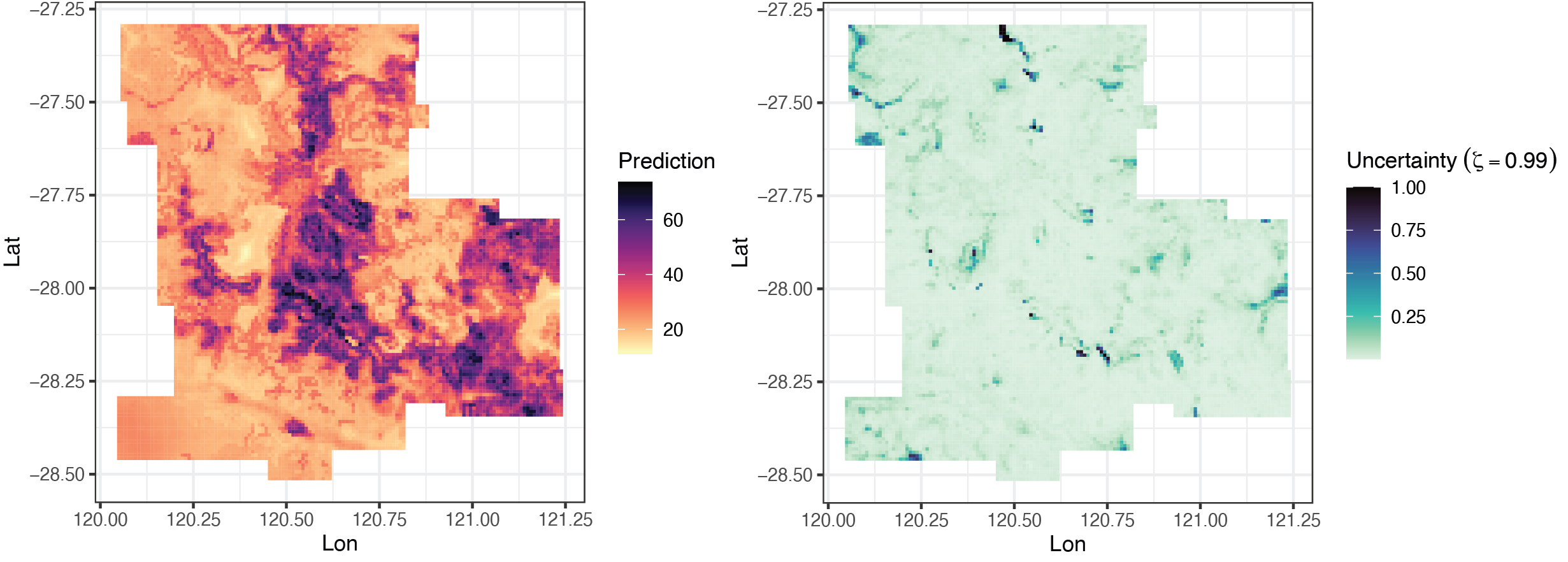# Geographically Optimal Similarity (GOS) and the Third Law of Geography in R

### Citation for package geosimilarity

To cite geosimilarity R package in publications, please use:

Song, Y. (2022) “Geographically Optimal Similarity”, Mathematical Geosciences. doi: 10.1007/s11004-022-10036-8.

## 1. Introduction to geosimilarity package

The package can be used to address following issues:

• Geographically optimal similarity (GOS) modeling.

• Modeling the Third Law of Geography (i.e., basic configuration similarity (BCS) model).

• Spatial prediction.

More details of GOS models can be found in Song (2022).

## 2. Spatial prediction using GOS model

According to Song (2022), GOS model consists of four primary steps: (1) Characterizing geographical configurations, (2) determining parameters for the optimal similarity, (3) spatial prediction using GOS and uncertainty assessment, and (4) model evaluation. The process of using geosimilarity package to conduct GOS modeling is presented as follows.

### 2.1 Characterizing geographical configurations

The geosimilarity package contains two spatial datasets:

• zn: Spatial samples of Zn concentrations and explanatory variables at sample locations

• grid: Spatial grid data of explanatory variables used for the prediction

install.packages("geosimilarity")
library("geosimilarity")
## This is geosimilarity 2.2.
##
## To cite geosimilarity in publications, please use:
##
## Song, Y. (2022). Geographically Optimal Similarity. Mathematical Geosciences. doi: 10.1007/s11004-022-10036-8.
## 
data("zn")
head(zn)
## # A tibble: 6 × 12
##     Lon   Lat    Zn Elevat…¹ Slope Aspect  Water  NDVI   SOC    pH    Road  Mine
##   <dbl> <dbl> <dbl>    <dbl> <dbl>  <dbl>  <dbl> <dbl> <dbl> <dbl>   <dbl> <dbl>
## 1  120. -28.5    10     455. 0.236  306.  0.014  0.184 0.909  5.95 49.4     55.6
## 2  120. -28.4    30     451. 0.207  293.  2.20   0.202 0.906  6.05 49.0     51.1
## 3  120. -28.4    30     443. 0.285  325.  0.0119 0.163 0.848  5.76 45.1     45.0
## 4  120. -27.4    30     509. 0.236   98.4 3.06   0.204 0.851  5.82  0.0774  49.0
## 5  120. -28.3    33     427. 0.191  329.  3.53   0.179 0.933  5.85 39.9     39.8
## 6  120. -27.3    27     510. 0.211  105.  3.38   0.191 0.868  6.07  0.0468  48.7
## # … with abbreviated variable name ¹​Elevation

Data pre-processing and variable selection:

# log-transformation
hist(zn$Zn) zn$Zn <- log(zn$Zn) hist(zn$Zn)

# remove outliers
library(SecDim)
k <- rmvoutlier(zn$Zn, coef = 2.5) dt <- zn[-k,] # correlation library("PerformanceAnalytics") cor_dt <- dt[, c(3:12)] chart.Correlation(cor_dt, histogram = TRUE, pch = 19) # multicollinearity library(car) m1 <- lm(Zn ~ Slope + Water + NDVI + SOC + pH + Road + Mine, data = dt) car::vif(m1) In this step, the selected variables include Slope, Water, NDVI, SOC, pH, Road, and Mine. ### 2.2 Determining the optimal similarity In the bestkappa function, if you set more optional numbers to the kappa vector and a higher value of the cross-validation repeat times nrepeat, a $$\kappa$$ value enabling more accurate prediction will be selected, but the computation time will be increased. For instance, if the optional kappa is (0.01, 0.05, 0.1, 0.2, 0.5, 1) and nrepeat is 2, the $$\lambda$$ (the optimal $$\kappa$$) is 0.10, and its corresponding mean cross-validation RMSE is 0.6591. The computation time is 5.9s. system.time({ # 5.9s b1 <- bestkappa(Zn ~ Slope + Water + NDVI + SOC + pH + Road + Mine, data = dt, kappa = c(0.01, 0.05, 0.1, 0.2, 0.5, 1), nrepeat = 2) }) b1$bestkappa
b1$cvmean b1$plot

If the optional kappa is (0.01, 0.02, …, 0.09, 0.1, 0.2, …, 1) and nrepeat is 10, the $$\lambda$$ (the optimal $$\kappa$$) is 0.08, and its corresponding mean cross-validation RMSE is 0.6668, which are more stable and reliable. The computation time is 97.7s.

system.time({ # 97.7ss
b2 <- bestkappa(Zn ~ Slope + Water + NDVI  + SOC + pH + Road + Mine,
data = dt,
kappa = c(seq(0.01, 0.1, 0.01), seq(0.2, 1, 0.1)),
nrepeat = 10)
})
b2$bestkappa b2$cvmean
b2$plotFigure 1. Processes of determining the optimal similarity. (a) The optional kappa is (0.01, 0.05, 0.1, 0.2, 0.5, 1) and nrepeat is 2. (b) The optional kappa is (0.01, 0.02, …, 0.09, 0.1, 0.2, …, 1) and nrepeat is 10. ### 2.3 Spatial prediction system.time({ # 26s g2 <- gos(Zn ~ Slope + Water + NDVI + SOC + pH + Road + Mine, data = dt, newdata = grid, kappa = 0.08) }) grid$pred <- exp(g2$pred) grid$uc99 <- g2$uncertainty$0.99
library(ggplot2)
library(viridis)
ggplot(grid, aes(x = Lon, y = Lat, fill = pred)) +
geom_tile() +
scale_fill_viridis(option="magma", direction = -1) +
coord_equal() +
labs(fill='Prediction') +
theme_bw()
ggplot(grid, aes(x = Lon, y = Lat, fill = uc99)) +
geom_tile() +
scale_fill_viridis(option="mako", direction = -1) +
coord_equal() +
labs(fill=bquote(Uncertainty~(zeta==0.99))) +
theme_bw()Figure 2. Geographially optimal similarity (GOS)-based prediction (a) and uncertainty (b).

In addition, the following codes can be used to plot uncertainty under different $$\zeta$$ values.

library(reshape2)
uc <- cbind(grid[,2:3], g2\$uncertainty)
uc <- melt(uc, id = c("Lon", "Lat"))
ggplot(uc, aes(x = Lon, y = Lat, fill = value)) +
geom_tile() +
scale_fill_viridis(option="mako", direction = -1) +
coord_equal() +
facet_wrap(~ variable) +
labs(fill='Uncertainty') +
theme_bw()